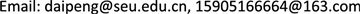1江苏方天电力技术有限公司，江苏 南京

2东南大学计算机科学与工程学院，江苏 南京1. 引言

2. 相关工作2.1. 学术搜索引擎

2.2. 基于实体的检索模型

3. 基于实体依赖建模的检索模型

3.1. MRF检索模型

P G , Λ ( Q , D ) = 1 Z Λ ∏ c ∈ C ( G ) ψ ( c ; Λ )

MRF模型的联合概率分布通常基于无向图上的最大团参数化，但用于检索模型时，这样的方式太过粗糙。这里为了在更细粒度的水平上将特征函数与团相关联，同时保持特征的数量，从而保持参数的数量。对于上述定义的三种类型的团，每个定义一种特征函数。

3.2. 检索框架

P G , Λ ( D , Q , E ) = 1 Z Λ ∏ c ∈ C ( G ) ψ ( c , Λ )

P G , Λ ( D | Q , E ) = P G , Λ ( Q , D , E ) P G , Λ ( Q , E ) ⇒ log P G , Λ ( Q , D , E ) − log P G , Λ ( Q , E ) ⇒ ∑ c ∈ C ( G ) log ψ ( c ; Λ ) (1)

P G , Λ ( D | Q , E ) = ( 1 − λ E ) ∑ c ∈ C w ( G ) log ψ w ( c ; Λ ) + λ E ∑ c ∈ C E ( G ) log ψ E ( c ; Λ ) (2)

3.3. 实体依赖模型

Entity dependency type definitio

T E包含文档节点和一个查询实体节点的团集。
O E包含文档节点和在查询中出现的多个(两个及以上)连续查询实体的团集。
U E包含文档节点和在查询中以任意顺序出现的多个(两个及以上)查询实体的团集。

O E 和 U E 都体现了实体的共现关系，但这里不使用 O E 团集，这是基于以下两个因素的考虑：1) 由于实体本身为一个独立语义单元，实体与实体之间序列依赖关系并不明显，一个常见的例子是对于多关键词罗列的查询，如“CRISPR/Cas9，ZFN，TALEN”，替换关键词之间的顺序，并不影响查询结果。2) 受限于实体识别精度，查询中识别出的实体并不完备，实体间可能有未识别出的实体。

3.4. 特征函数定义

f T E ( e i , d j ) = log P ( e i | d j ) = log ∑ m = 1 k w m T ⋅ P ( e i | d j m )

P ( e i | d j m ) = n e i , d j m + u m T n e i , D m L D m L d j m + u m T

n e i , d j m 表示实体 e i 出现在 d j m 的次数， L d j m 表示文档j字段m的总长度。与 和 定义类似， n e i , D m 和 L D m 为在文档集合上的定义， u m T 是字段j的狄利克雷平滑因子  。

f U E ( e i , i + 1 , d j ) = log P ( e i , e i + 1 | d j ) = log ∑ m = 1 k w m U ⋅ P ( e i , e i + 1 | d j m )

P ( e i , e i + 1 | d j m ) = n e i , i + 1 , d j m + u m n e i , i + 1 , D m L D m L d j m + u m

g ( t ) = 1 + log ( N D n t )

f T E ( e i , d j ) = g ( e i ) ⋅ log ∑ m = 1 k w m T ⋅ P ( e i | d j m ) (3)

f U E ( e i , i + 1 , d j ) = g ( e i , e i + 1 ) ⋅ log ∑ m = 1 k w m U ⋅ P ( e i , e i + 1 | d j m ) (4)

3.5. 实体类型信息

· Process (P)：表示与某些科学模型，算法或过程相关的科学概念实体。

· Other (O)：表示除上述定义的两种类型实体外，文献中涉及的其它科学概念实体。

biLSTM-CRF模型在进行NER任务时，利用上下文信息，编码了文本的词法和语法信息，因此处理短句子或者不完整文本描述效果并不好，适合于文献标题、摘要、正文等长文本数据的实体识别。对于查询上实体类别信息的识别，本文基于伪相关反馈策略对查询中实体类型进行估计。具体的，首先利用公式(2)计算与用户查询的相关文档，然后基于相关文档，我们定义查询实体类型的概率分布为：

P ( t | E , D , Q ) ∝ P ( t | D ) P ( D | Q , E ) ≈ ∑ d i ∈ D R P ( t | d i ) P ( d i | Q , E )

D R 表示与查询相关的TOP-K的相关文档集合，t表示实体类型， t ∈ { T , P , O } 。

n e i , d j m = ∑ e i ∈ E q ∑ e j ∈ E d m a t c h ( e i , e j )

m a t c h ( e i , e j ) = { 1                   t y p e ( e i ) ≠ t y p e ( e j ) ∧ e i = e j 0                                             e i ≠ e j η                 t y p e ( e i ) = t y p e ( e j ) ∧ e i = e j

4. 实验4.1. 实验数据集

ESR数据集的查询是从学术搜索引擎Semantic Scholar在2016的查询日志中采样构成。总共包含100个查询，其中20个采样自高频查询，30来自中频查询，剩余50个采样自Semantic Scholar表现较差的困难查询，主要涉及计算机科学领域。查询候选文档是从Semantic Scholar在线搜索系统汇集生成，共有8541篇候选文档，每个相关文档都由人工标记，共分5-level的相关度。

4.2. 实体识别

4.3. 检索性能

Comparison of BM25, QL and EBMRF retrieval result
NDCG@20P@20
BM25-W0.35540.2977
BM25-E0.32320.2742
BM25-Dual0.36860.3075
QL-W0.34970.2916
QL-E0.31850.2612
QL-Dual0.35980.2998
EBMRF-W0.37350.3106
EBMRF-E0.34990.2901
EBMRF-Dual0.3924 0.3315

Comparison of retrieval results between EBMRF and EBMRF+ on multi-entity querie
ESRESR-ME
NDCG@20P@20NDCG@20P@20
EBMRF0.39240.33150.4468 0.3761
EBMRF+ 0.38190.32070.42010.3568

Comparison of retrieval results between EBMRF and EBMRF
ESR
NDCG@5NDCG@10NDCG@20
EBMRF0.32450.35020.3924
EBMRF* 0.33810.35860.3966
P@5P@10P@20
EBMRF0.44830.39780.3315
EBMRF* 0.47040.40460.3288

5. 总结